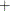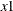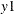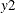# The QLIM Procedure

### RESTRICT Statement

• RESTRICT restriction1 <, restriction2 …> ;

The RESTRICT statement is used to impose linear restrictions on the parameter estimates. Any number of RESTRICT statements can be specified, but the number of restrictions imposed is limited by the number of regressors.

Each restriction is written as an expression, followed by an equality operator (=) or an inequality operator (<, >, <=, >=), followed by a second expression:

• expression operator expression

The operator can be =, <, >, <= , or >=. The operator and second expression are optional.

Restriction expressions can be composed of parameter names, multiplication (), addition () and substitution () operators, and constants. Parameters named in restriction expressions must be among the parameters estimated by the model. Parameters associated with a regressor variable are referred to by the name of the corresponding regressor variable. The restriction expressions must be a linear function of the parameters.

The following is an example of the use of the RESTRICT statement:

```   proc qlim data=one;
model y = x1-x10 / discrete;
restrict x1*2 <= x2 + x3;
run;
```

The RESTRICT statement can also be used to impose cross-equation restrictions in multivariate models. The following RESTRICT statement imposes an equality restriction on coefficients ofin equationandin equation:

```   proc qlim data=one;
model y1 = x1-x10;
model y2 = x1-x4;
endogenous y1 y2 ~ discrete;
restrict y1.x1=y2.x1;
run;
```

The RESTRICT statement is not supported if a BAYES statement is also specified. In Bayesian analysis, the restrictions on parameters are usually introduced through the prior distribution.